Practice Set 6.1 Statistics Class 10th Mathematics Part 1 MHB Solution

Practice Set 6.1

1. The following table shows the number of students and the time they utilized daily for…
2. In the following table, the toll paid by drivers and the number of vehicles is shown.…
3. A milk centre sold milk to 50 customers. The table below gives the number of customers…
4. A frequency distribution table for the production of oranges of some farm owners is…
5. A frequency distribution of funds collected by 120 workers in a company for the drought…
6. The following table gives the information of frequency distribution of weekly wages of…

Practice Set 6.1

Question 1.

The following table shows the number of students and the time they utilized daily for their studies. Find the mean time spent by students for their studies by direct method.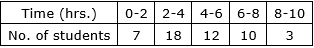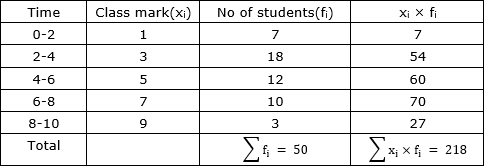⇒ Mean =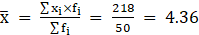⇒ Mean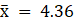Question 2.

In the following table, the toll paid by drivers and the number of vehicles is shown. Find the mean of the toll by 'assumed mean' method.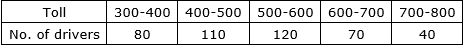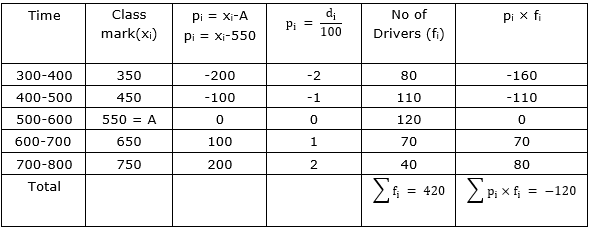⇒ Mean =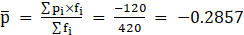⇒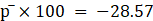⇒ Mean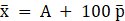⇒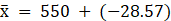⇒ Mean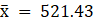Mean toll is Rs 521.43

Question 3.

A milk centre sold milk to 50 customers. The table below gives the number of customers and the milk they purchased. Find the mean of the milk sold by direct method.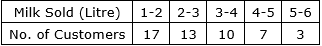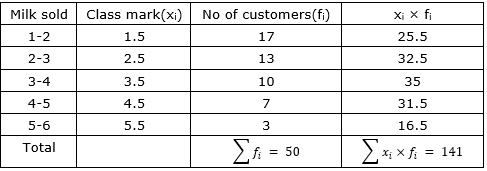⇒ Mean =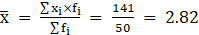litre

⇒ Mean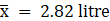Mean of the milk sold is 2.82 litre

Question 4.

A frequency distribution table for the production of oranges of some farm owners is given below. Find the mean production of oranges by 'assumed mean' method.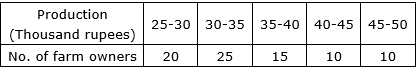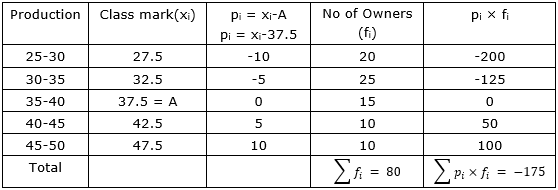⇒Mean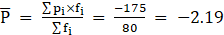Mean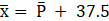= 37.5-2.19

= 35.31

⇒The amount is given in thousands or rupees

∴ 35.31× 1000 = Rs 35130

Question 5.

A frequency distribution of funds collected by 120 workers in a company for the drought affected people are given in the following table. Find the mean of the funds by 'step deviation' method.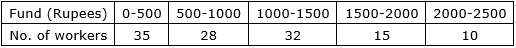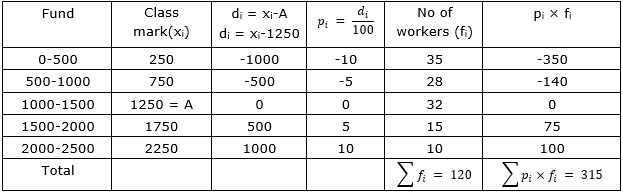⇒ Mean =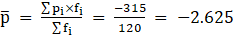⇒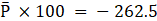⇒ Mean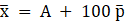⇒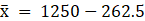⇒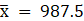∴Mean of the funds is Rs 987.5

Question 6.

The following table gives the information of frequency distribution of weekly wages of 150 workers of a company. Find the mean of the weekly wages by 'step deviation' method.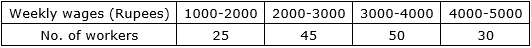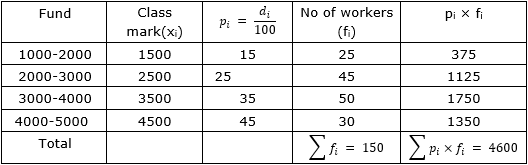Mean =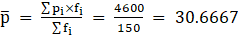⇒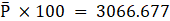⇒ Mean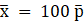⇒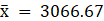∴ Mean of weekly wages is Rs 3066.67